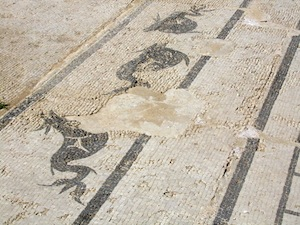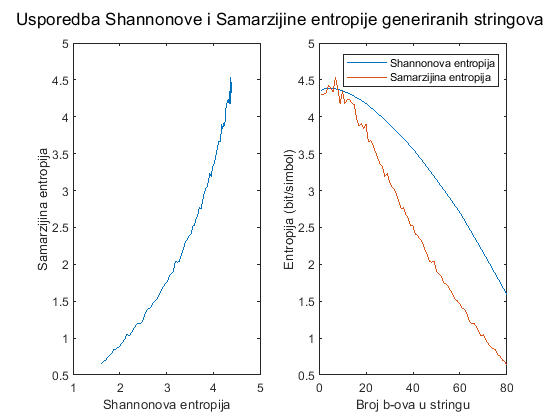Croatian Toponyms

Toponyms are often affected by the linguistic phenomenon called tautology. A toponym is often composed of more words in different languages meaning exactly the same. A famous example of that is Torpenhow Hill.

# My Interpretation of the Croatian ToponymsThe Salt Lake
on the Mljet Island.
It's sometimes suggested
that the islands
were once the places richest
in toponyms, because people
every single source of
fresh water and every single
piece of fertile land.

I was asked to create a web-page in which I summarize my alternative interpretation of the Croatian toponyms, which I have supported on many Internet forums and on some conferences (full text is available in this PDF on the page 70), so that we have everything about it on one page. Here we go!

ATTENTION: Some of the opinions stated in the following text are contrary to the mainstream science. I will not advise you to read it if you don't have a substantial background in linguistics. I am not a conspiracy theorist who wants to bombard people with controversial statements they don't know how to evaluate, and I am not denying it is possible my work is to historical linguistics what Anatoly Fomenko's work is to history. If you are ready to read it, click here.The Roman Thermae
in Issa (Vis)
were getting the water
from a mineral spring
that doesn't exist
any more.
However, it's possible
that Issa was
named after it,
from the Indo-European
root *yos (spring).

That would be it! If you want to discuss my theory, go to the "Croatian Toponyms" forum thread I've linked to on the left. I'd like to have some sane opposition there, because I think my interpretation may be right. Ideas are correct or incorrect independent of their creators. The fact that I am not a linguist specializing in those things doesn't mean my ideas are wrong. I've used the methods that are well-accepted in linguistics (apart from applying statistics to the toponyms, which is for some reason very rarely done), I've just come to the conclusions that are different from the mainstream ones.
UPDATE on 09/07/2018: You can download my Illyrian-Croatian dictionary here (it's a .DOCX file!).
UPDATE on 11/04/2021: I managed to install MatLab on my computer. So, here is that Octave program related to entropies modified so that it can be run in MatLab:
```% Ovo je MatLabski program koji uspoređuje rezultate koje daje moj algoritam
% procjenjivanja entropije s rezultatima koje daje Shannonov algoritam.
suglasnici = 'bcdfghjklmnpqrstvwxyz';
testni_stringovi=cell(100 - length(suglasnici) + 1, 1);
for koliko_cemo_staviti_b_ova = 100 - length(suglasnici) + 1 : -1 : 1
for i = 1 : koliko_cemo_staviti_b_ova
testni_stringovi{koliko_cemo_staviti_b_ova} = [
testni_stringovi{koliko_cemo_staviti_b_ova} 'b'
];
end
for i = 1 : 100 - koliko_cemo_staviti_b_ova
testni_stringovi{koliko_cemo_staviti_b_ova} = [
testni_stringovi{koliko_cemo_staviti_b_ova} suglasnici(int32(floor((i - 1) / (100 - koliko_cemo_staviti_b_ova) * (length(suglasnici) - 1))) + strfind(suglasnici, 'c'))
];
end
end
samarzijine_entropije = [];
shannonove_entropije = [];
for i = 1 : length(testni_stringovi)
str = testni_stringovi{i};
samarzijine_entropije = [samarzijine_entropije samarzijina_entropija(str)];
shannonove_entropije = [shannonove_entropije shannonova_entropija(str, suglasnici)];
end
sgtitle('Usporedba Shannonove i Samarzijine entropije generiranih stringova');
subplot(1,2,1);
plot(shannonove_entropije, samarzijine_entropije);
xlabel('Shannonova entropija');
ylabel('Samarzijina entropija');
subplot(1,2,2);
plot(shannonove_entropije);
hold on;
plot(samarzijine_entropije);
xlabel('Broj b-ova u stringu');
ylabel('Entropija (bit/simbol)');
legend('Shannonova entropija', 'Samarzijina entropija');
function ret = shannonova_entropija(str, suglasnici)
apsolutne_frekvencije = [];
for i = 1 : length(suglasnici)
apsolutne_frekvencije = [apsolutne_frekvencije 0];
end
for i = 1 : length(str)
znak = str(i);
apsolutne_frekvencije(strfind(suglasnici, znak)) = apsolutne_frekvencije(strfind(suglasnici, znak)) + 1;
end
relativne_frekvencije = apsolutne_frekvencije / length(str);
ret = 0;
for relativna_frekvencija = relativne_frekvencije
if relativna_frekvencija > 0
ret = ret - log2(relativna_frekvencija) * relativna_frekvencija;
end
end
end
function ret = samarzijina_entropija(str)
broj_pokusaja = 10000;
broj_pogodaka = 0;
for i = 1 : broj_pokusaja
prvi = int32(floor(rand() * length(str) + 1));
drugi = int32(floor(rand() * length(str) + 1));
if str(prvi) == str(drugi)
broj_pogodaka = broj_pogodaka + 1;
end
end
omjer_pogodaka = broj_pogodaka / broj_pokusaja;
ret = -log2(omjer_pogodaka);
end```
Here is what it outputs:UPDATE on 14/04/2021: I have found out why the Octave program and the MatLab program give wildly different results. Namely, there was a syntax error in my program. MatLab refused to parse it, but Octave was apparently doing automatic semicolon insertion (like JavaScript engines are doing). That resulted in incorrect test strings in `testni_stringovi`. This is what the test strings look like in MatLab when exported to a CSV file, and this is what they look like when exported from Octave.
Anyway, since we know the number of possible consonant pairs in Croatian is 26*26=676, the maximal possible entropy a consonant pair in the Croatian language could have is log2(676)=9.4 bits/symbol. And we have measured the Shannon's entropy to be log2(229)=7.839. So, assuming the curve representing the relationship between the Samaržija's entropy and the Shannon's entropy does not change its shape between individual consonants and consonants pairs, but only scales uniformly (which I have no idea how to test), we can estimate the Samaržija's entropy of the consonant pairs in the Croatian language the following way. We can assume the entropy of the pairs of consonants is log2(676)/log2(21)=2.14 times bigger than the corresponding entropy of individual consonants. The ratio between the measured Shannon's entropy and the maximal possible entropy in this case is 7.839/9.4=0.834. Thus, the corresponding point on the curve on the above diagram is when the Shannon's entropy is equal to 0.834*log2(21)=3.663 bits/symbol. The Samaržija's entropy at that point, as can be read from the diagram, is around 2.8 bits/symbol. Thus, we can expect the Samaržija's entropy of the pairs of consonants in Croatian to be around 2.14*2.8=5.992 bits/symbol. Thus, the probability of two random words beginning with the same pair of consonants should be around 1/(2^5.992)=1/63.65=1.57%. If that is true, then the p-value of that pattern of the Croatian river names starting with *karr~kurr is only 5.9% (the highest estimate I got by running the birthday-paradox-calculation written in C a few times), rather than around 1/500. Well, I guess it is always like that in social sciences: If you think you have a good p-value, you are probably calculating something incorrectly.

UPDATE on 29/04/2021: You can see the draft of the next paper about linguistics I am planning to publish.